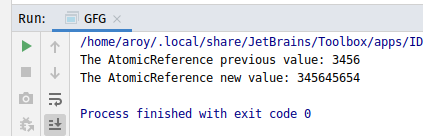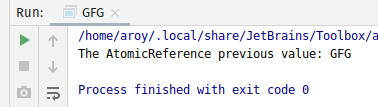# AtomicReference getAndAccumulate() method in Java with Examples

The getAndAccumulate() method of a AtomicReference class is used to atomically updates the current value of AtomicReference with the results of applying the given accumulatorFunction to the current and given values and returns the previous value. The accumulatorFunction should be side-effect-free, since it may be re-applied when attempted updates fail due to contention among threads. The function is applied with the current value as its first argument, and the given update as the second argument.

Syntax:

```public final E getAndAccumulate(E x,
BinaryOperator<E> accumulatorFunction)

```

Parameters: This method accepts:

• x which is the updated value and
• accumulatorFunction which is a side-effect-free function of two arguments.

Return value: This method returns the prevoius value.

Below programs illustrate the getAndAccumulate() method:
Program 1:

 `// Java program to demonstrate ` `// AtomicReference.getAndAccumulate() method ` ` `  `import` `java.util.concurrent.atomic.*; ` `import` `java.util.function.BinaryOperator; ` ` `  `public` `class` `GFG { ` `    ``public` `static` `void` `main(String args[]) ` `    ``{ ` ` `  `        ``// AtomicReference with value ` `        ``AtomicReference ref ` `            ``= ``new` `AtomicReference<>(``3456``); ` ` `  `        ``// Value to apply getAndAccumulate ` `        ``int` `x = ``45654``; ` ` `  `        ``// Declaring the accumulatorFunction ` `        ``// applying function to add value as string ` `        ``BinaryOperator add ` `            ``= (u, v) -> u.toString() + v.toString(); ` ` `  `        ``// apply getAndAccumulate() ` `        ``int` `value = ref.getAndAccumulate(x, add); ` ` `  `        ``// print AtomicReference ` `        ``System.out.println( ` `            ``"The AtomicReference previous value: "` `            ``+ value); ` `        ``System.out.println( ` `            ``"The AtomicReference new value: "` `            ``+ ref.get()); ` `    ``} ` `} `

Output:Program 2:

 `// Java program to demonstrate ` `// AtomicReference.getAndAccumulate() method ` ` `  `import` `java.util.concurrent.atomic.*; ` `import` `java.util.function.BinaryOperator; ` ` `  `public` `class` `GFG { ` `    ``public` `static` `void` `main(String args[]) ` `    ``{ ` `        ``// AtomicReference with value ` `        ``AtomicReference ref ` `            ``= ``new` `AtomicReference(``"GFG "``); ` ` `  `        ``// Value to apply getAndAccumulate ` `        ``String x = ``"Welcome"``; ` ` `  `        ``// Declaring the accumulatorFunction ` `        ``// applying function to add value as string ` `        ``BinaryOperator add ` `            ``= (u, v) -> v + ``" to "` `+ u; ` ` `  `        ``// apply getAndAccumulate() ` `        ``String previousValue ` `            ``= ref.getAndAccumulate(x, add); ` ` `  `        ``// print AtomicReference ` `        ``System.out.println( ` `            ``"The AtomicReference previous value: "` `            ``+ previousValue); ` `    ``} ` `} `

Output:Don’t stop now and take your learning to the next level. Learn all the important concepts of Data Structures and Algorithms with the help of the most trusted course: DSA Self Paced. Become industry ready at a student-friendly price.

My Personal Notes arrow_drop_upCheck out this Author's contributed articles.

If you like GeeksforGeeks and would like to contribute, you can also write an article using contribute.geeksforgeeks.org or mail your article to contribute@geeksforgeeks.org. See your article appearing on the GeeksforGeeks main page and help other Geeks.

Please Improve this article if you find anything incorrect by clicking on the "Improve Article" button below.

Article Tags :
Practice Tags :

Be the First to upvote.

Please write to us at contribute@geeksforgeeks.org to report any issue with the above content.